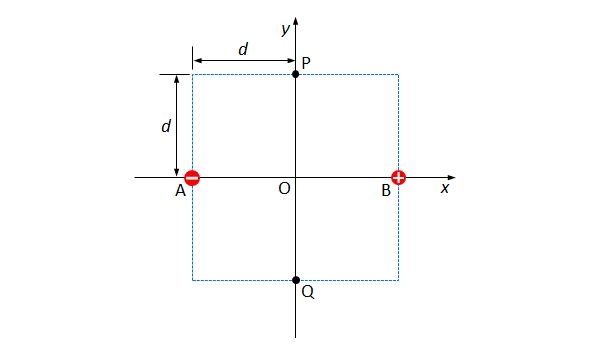# Point charges in planeAs shown above, two point charges $A$ and $B$ are fixed on the $x$-axis with an equal distance of $d$ from the origin $O.$ $A$ is charged negatively, while $B$ is charged positively. The quantities of the electric charges on $A$ and $B$ are the same. The distances between the origin $O$ and the two points $P$ and $Q$ on the $y$-axis are also the same, which is $d.$ Which of the following is correct?

a) The electric field intensities at $P$ and $Q$ are the same.

b) The direction of the electric field at $O$ is the $-x$ direction.

c) If we move the point charge $A$ to $O$, then the directions of the electric fields at $P$ and $Q$ are the same.

×

Problem Loading...

Note Loading...

Set Loading...